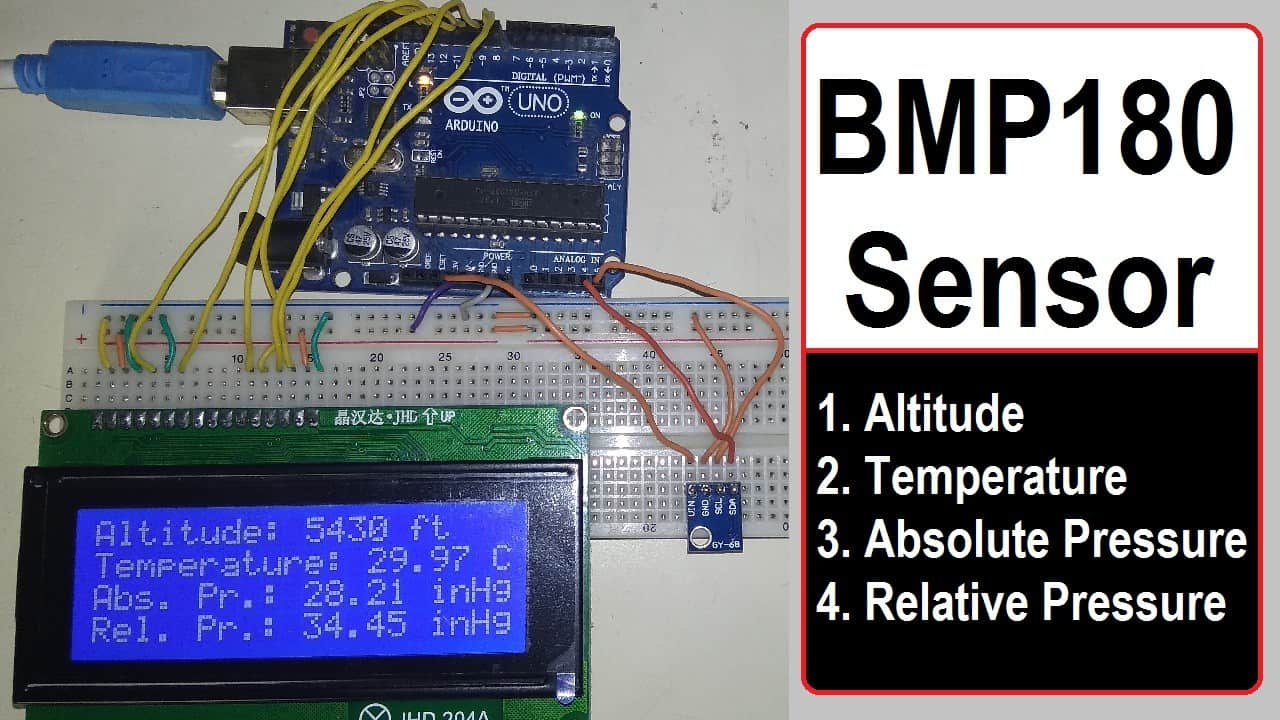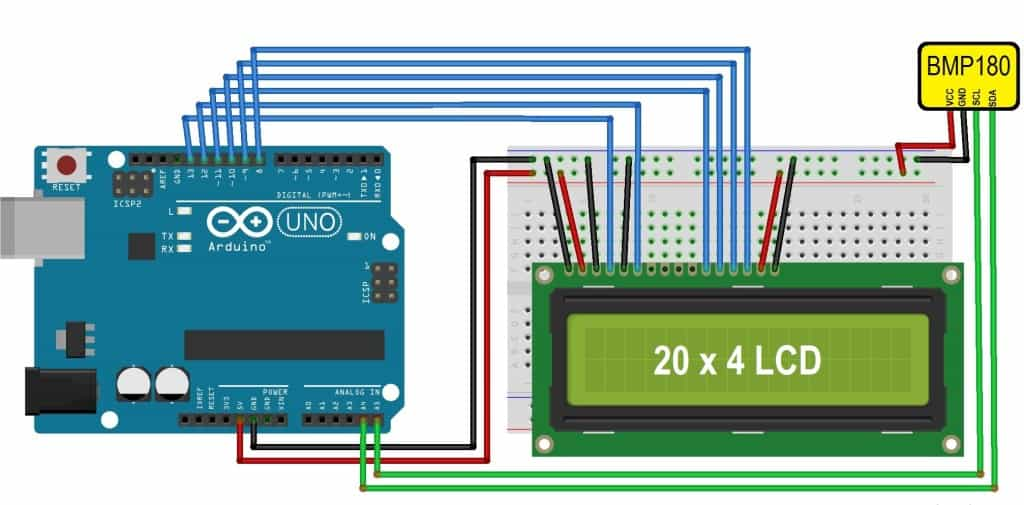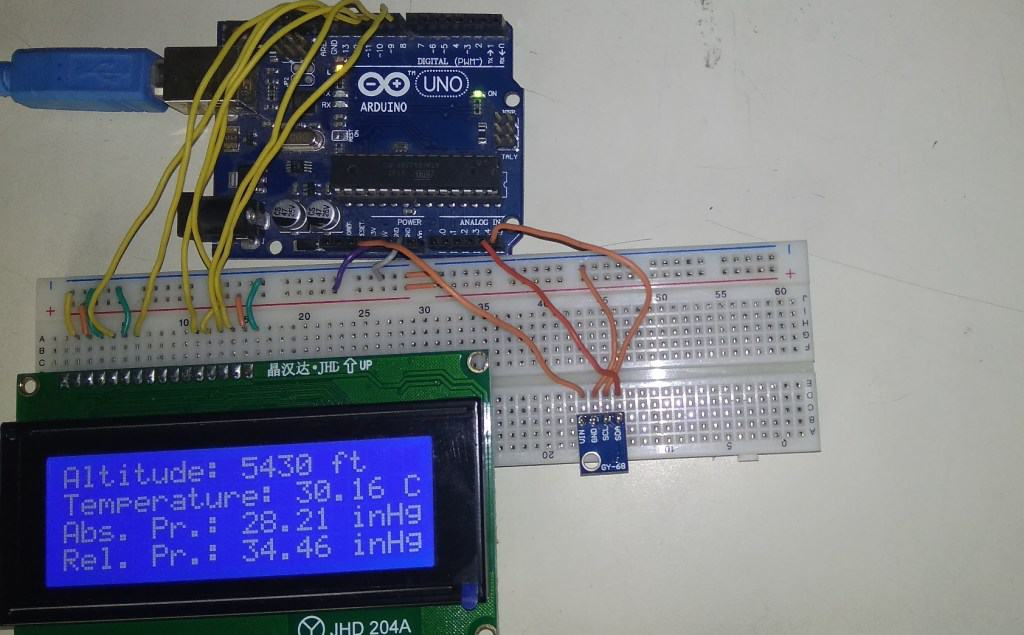BMP180 for Altitude, Pressure & Temperature Measurement using Arduino(Last Updated On: February 25, 2019)

Introduction:

In this post we will learn how to Interface BMP180 Sensor with Arduino to measure Altitude, Pressure & Temperature using Arduino. Here we will learn about the barometric sensor BMP180 for Altitude, Pressure & Temperature Measurement using Arduino. The BMP180 Sensor is a barometric sensor capable of measuring pressure, temperature & altitude as well. The BMP180 Arduino Code is given below. But before that you can go through our easy tutorial about BMP180 through this link:How to interface BMP180 with Arduino

With Arduino Barometric condition is one of the criteria used to predict coming change in weather and deduce altitude above sea level. Here is a demo to show you how to read the barometric data from this Grove – Barometer Sensor (BMP180).BMP180 for Altitude, Pressure & Temperature Measurement using Arduino:

Components Required:

```1. Arduino UNO Board
2. BMP180 Bosch Sensor
3. 16 x 2 LCD
5. Connecting wires```

Circuit Diagram:BMP180 Barometric Sensor:

Introduction:

This precision sensor from Bosch is the best solution for measuring barometric pressure and temperature. It is an ultra low-power digital temperature and pressure sensor with high accuracy and stability. Because pressure changes with altitude you can also use it as an altimeter. It measures the absolute pressure of the air around it. It has a measuring range from 300 to 1100hPa with an accuracy down to 0.02 hPa. It can also measure altitude and temperature.It consists of a piezo-resistive sensor, an analog to digital converter and a control unit with EEPROM and a serial I2C interface. The raw measurements of pressure and temperature from the BMP180 sensor has to be compensated for temperature effects and other parameters using the calibration data saved into the EEPROM.

Here we will Interface BMP180 Sensor with Arduino. We will use an Arduino board to read the temperature and barometric pressure measurements from the BMP180 sensor and display the data on 16*2 LCD.

BMP180 Features & Specifications:

```1. Supply Voltage:1.8V to 3.6V
2. Low power consumption:0.5uA at 1Hz
3. I2C interface
4. Max I2C Speed: 3.5Mhz
5. Very low noise: up to 0.02hPa (17cm)
6. Pressure Range: 300hPa to 1100hPa (+9000m to -500m)```

Pin Details:

1. Vin – +5V DC
2. GND – GND
3. SCL – I2C Interface
4. SDA – I2C Interface

Working of BMP180:

The BMP180 consists of a piezo-resistive sensor, an analog to digital converter and a control unit with E2PROM and a serial I2C interface. The BMP180 delivers the uncompensated value of pressure and temperature. The microcontroller sends a start sequence to start a pressure or temperature measurement. After converting time, the result value (pressure or temperature respectively) can be read via the I2C interface.For calculating temperature in °C and pressure in hPa (hecto Pascal), the calibration data has to be used. These constants can be read out from the BMP180 E2PROM via the I2C interface at software initialization. The sampling rate can be increased up to 128 samples per second (standard mode) for dynamic measurement. In this case, it is sufficient to measure the temperature only once per second and to use this value for all pressure measurements during the same period.

Working of the Project:This project BMP180 for Altitude, Pressure & Temperature Measurement using Arduino can be explained via program functions and variables.

Like most pressure sensors, the BMP180 measures absolute pressure. This is the actual ambient pressure seen by the device, which will vary with both altitude and weather. Before taking a pressure reading you must take a temperature reading. This is done with startTemperature() and getTemperature(). The result is in degrees C. Once you have a temperature reading, you can take a pressure reading. This is done with startPressure() and getPressure(). The result is in millibar (mb) aka hectopascals (hPa).

If you’ll be monitoring weather patterns, you will probably want to remove the effects of altitude. This will produce readings that can be compared to the published pressure readings from other locations. To do this, use the sealevel() function. You will need to provide the known altitude at which the pressure was measured.

If you want to measure altitude, you will need to know the pressure at a baseline altitude. This can be average sealevel pressure, or a previous pressure reading at your altitude, in which case subsequent altitude readings will be + or – the initial baseline. This is done with the altitude() function.

Since pressure varies with altitude, you can use a pressure sensor to measure altitude (with a few caveats). The average pressure of the atmosphere at sea level is 1013.25 hPa (or mbar). This drops off to zero as you climb towards the vacuum of space. Because the curve of this drop-off is well understood, you can compute the altitude difference between two pressure measurements (p and p0) by using this equation:Program/Source Code:

To interface BMP180 Sensor with Arduino to measure Altitude, Pressure & Temperature copy the code below and upload it to the Arduino UNO Board. the code given below is for the project BMP180 for Altitude, Pressure & Temperature Measurement using Arduino.

```#include <LiquidCrystal.h>
LiquidCrystal lcd(13, 12, 11, 10, 9, 8);//RS,EN,D4,D5,D6,D7
#include <SFE_BMP180.h>
#include <Wire.h>
// You will need to create an SFE_BMP180 object, here called "pressure":
SFE_BMP180 pressure;
#define ALTITUDE 1655.0 // Altitude of SparkFun's HQ in Boulder, CO. in meters
void setup()
{
Serial.begin(9600);
Serial.println("BMP180 Measurements");
lcd.begin(20, 4);
lcd.setCursor(0, 0);
lcd.print("BMP180 Measurements");
lcd.setCursor(0, 1);
lcd.print(" 1. Temperature");
lcd.setCursor(0, 2);
lcd.print(" 2. Pressure");
lcd.setCursor(0, 3);
lcd.print(" 3. Altitude");
delay (5000);
lcd.clear();//clear display
// Initialize the sensor (it is important to get calibration values stored on the device).
if (pressure.begin())
Serial.println("BMP180 init success");
else
{
// Oops, something went wrong, this is usually a connection problem,
// see the comments at the top of this sketch for the proper connections.
Serial.println("BMP180 init fail\n\n");
while(1); // Pause forever.
}
}
void loop()
{
char status;
double T,P,p0,a;
// Loop here getting pressure readings every 10 seconds.
// If you want sea-level-compensated pressure, as used in weather reports,
// you will need to know the altitude at which your measurements are taken.
// We're using a constant called ALTITUDE in this sketch:
Serial.println();
Serial.print("provided altitude: ");
lcd.setCursor(0, 0);
lcd.print("Altitude: ");
Serial.print(ALTITUDE,0);
Serial.print(" meters, ");
Serial.print(ALTITUDE*3.28084,0);
lcd.print(ALTITUDE*3.28084,0);
Serial.println(" feet");
lcd.print(" ft");
// If you want to measure altitude, and not pressure, you will instead need
// to provide a known baseline pressure. This is shown at the end of the sketch.
// You must first get a temperature measurement to perform a pressure reading.
// Start a temperature measurement:
// If request is successful, the number of ms to wait is returned.
// If request is unsuccessful, 0 is returned.
status = pressure.startTemperature();
if (status != 0)
{
// Wait for the measurement to complete:
delay(status);
// Retrieve the completed temperature measurement:
// Note that the measurement is stored in the variable T.
// Function returns 1 if successful, 0 if failure.
status = pressure.getTemperature(T);
if (status != 0)
{
// Print out the measurement:
Serial.print("temperature: ");
Serial.print(T,2);
Serial.print(" deg C, ");
Serial.print((9.0/5.0)*T+32.0,2);
Serial.println(" deg F");
lcd.setCursor(0, 1);
lcd.print("Temperature: ");
lcd.print(T,2);
lcd.print(" C ");
// Start a pressure measurement:
// The parameter is the oversampling setting, from 0 to 3 (highest res, longest wait).
// If request is successful, the number of ms to wait is returned.
// If request is unsuccessful, 0 is returned.
status = pressure.startPressure(3);
if (status != 0)
{
// Wait for the measurement to complete:
delay(status);
// Retrieve the completed pressure measurement:
// Note that the measurement is stored in the variable P.
// Note also that the function requires the previous temperature measurement (T).
// (If temperature is stable, you can do one temperature measurement for a number of pressure measurements.)
// Function returns 1 if successful, 0 if failure.
status = pressure.getPressure(P,T);
if (status != 0)
{
// Print out the measurement:
Serial.print("absolute pressure: ");
Serial.print(P,2);
Serial.print(" mb, ");
Serial.print(P*0.0295333727,2);
Serial.println(" inHg");
lcd.setCursor(0, 2);
lcd.print("Abs. Pr.: ");
lcd.print(P*0.0295333727,2);
lcd.print(" inHg");
// The pressure sensor returns abolute pressure, which varies with altitude.
// To remove the effects of altitude, use the sealevel function and your current altitude.
// This number is commonly used in weather reports.
// Parameters: P = absolute pressure in mb, ALTITUDE = current altitude in m.
// Result: p0 = sea-level compensated pressure in mb
p0 = pressure.sealevel(P,ALTITUDE); // we're at 1655 meters (Boulder, CO)
Serial.print("relative (sea-level) pressure: ");
Serial.print(p0,2);
Serial.print(" mb, ");
Serial.print(p0*0.0295333727,2);
Serial.println(" inHg");
lcd.setCursor(0, 3);
lcd.print("Rel. Pr.: ");
lcd.print(p0*0.0295333727,2);
lcd.print(" inHg");
// On the other hand, if you want to determine your altitude from the pressure reading,
// use the altitude function along with a baseline pressure (sea-level or other).
// Parameters: P = absolute pressure in mb, p0 = baseline pressure in mb.
// Result: a = altitude in m.
a = pressure.altitude(P,p0);
Serial.print("computed altitude: ");
Serial.print(a,0);
Serial.print(" meters, ");
Serial.print(a*3.28084,0);
Serial.println(" feet");
}
else Serial.println("error retrieving pressure measurement\n");
}
else Serial.println("error starting pressure measurement\n");
}
else Serial.println("error retrieving temperature measurement\n");
}
else Serial.println("error starting temperature measurement\n");
delay(5000); // Pause for 5 seconds.
}```

BMP180 Sensor Accuracy & Limitations:

1. Accuracy: How accurate is this? The theoretical noise level at the BMP180s highest resolution is 0.25m (about 10 inches), though in practice we see noise on the order of 1m (40 inches). You can improve the accuracy by taking a large number of readings and averaging them, although this will slow down your sample rate and response time.

2. Weather: You should also remember that pressure changes due to weather will affect your altitude readings. The best accuracy will be obtained if you take a “fresh” p0 when you need it and don’t rely on it to be accurate for extended periods due to changes in the weather.

3. Maximum altitude: The BMP180 can’t measure all the way down to vacuum (or up to space). It’s advertised lower limit is about 300 hPa (or mbar), which corresponds to an altitude of about 3000m or 30,000 feet. People have flown these to higher altitudes and gotten useful results, but this isn’t guaranteed or likely to be accurate. (You might consider using GPS for high-altitude measurements).

4. Minimum altitude: Similarly, this sensor isn’t suited for large pressures either. The advertised upper limit is 1100 hPa=mbar (or 16 psi), which is about 500 feet below sea level (that’s in air – the BMP180 isn’t submersible in water). This sensor isn’t a good choice for submersible or compressed-gas measurements.

Video Preview & Explanation:

BMP180 for Altitude, Pressure & Temperature Measurement using Arduino

Recommended For You